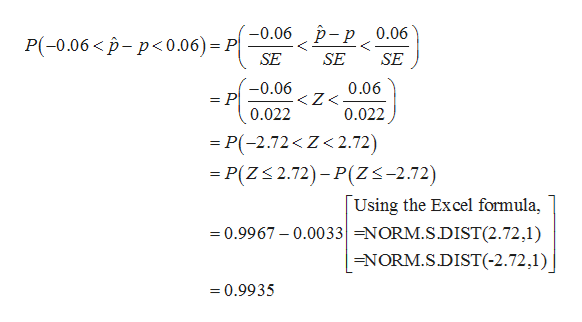# Suppose 47% of the population has a college degree.If a random sample of size 501 is selected, what is the probability that the proportion of persons with a college degree will differ from the population proportion by less than 6%? Round your answer to four decimal places.

Question
1 views

Suppose 47% of the population has a college degree.

If a random sample of size 501 is selected, what is the probability that the proportion of persons with a college degree will differ from the population proportion by less than 6%? Round your answer to four decimal places.

check_circle

Step 1

It is given that 47% of the population has a college degree. A sample of 501 is considered.

That is, p = 0.47 and n = 501.

The probability that the proportion of persons with a college degree will differ from the population proportion by less than 6% is P [-0.06 < (p-cap) – p < 0.06].

The required probability is, P [-0.06 < (p-cap) – p < 0.06].

The standard error required for further calculations is as shown below:

Step 2

The required probability can be standardized by diving with S...help_outlineImage Transcriptionclose-0.06 p- p 0.06 P(-0.06< p-p<0.06)= P| SE SE SE -0.06 = P 0.022 0.06

### Want to see the full answer?

See Solution

#### Want to see this answer and more?

Solutions are written by subject experts who are available 24/7. Questions are typically answered within 1 hour.*

See Solution
*Response times may vary by subject and question.
Tagged in

### Other